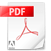How to measure bearings on a nautical chart:

How to measure distance on a nautical chart:

How to interpret tidal diamonds on a nautical chart:

# Introduction to Nautical Charts:

### What to include when teaching an intro?

• Chart Type/s
• Chart Name/title & year
• Colours
• Important Symbols - buoyage / hazards etc
• Land Marks (towers, lighthouses etc)
• Scale
• Latitude and Longitude
• Chart Datum
• Compass Rose
• Tidal Diamonds
• Text information sections

# Measuring Distance on a Nautical Chart:

Distances at sea are measured in nautical miles

1’ (minute) of latitude = 1 nautical mile

1∘ degree of Latitude = 60’ (minute) or 60 nautical miles

Land mile = 1609m

Nautical mile = 1852m / 1.15 land miles

Some charts (i.e. Imray) have a ‘Scale’ on the chart but if this is not available then you will need to measure the distance from the latitude scale (side) of the chart

### Why do we measure from the side (Latitude Scale) of the Chart?

Distances are always measuring from the Latitude Scale on the Chart NOT the Longitude Scale. As detailed in the image below, the distances between each latitude line are equal, whereas the distances between each Longitude line gets smaller as the lines approach the poles. Only at the Equator are the lines of latitude and longitude at the same distance.

### How to measure distance?

Measure the distance with your dividers, parallel rules, a regular ruler or even a piece of paper!

Take that distance to the latitude scale on the chart to gather the distance in minutes.

### How to work out the time it takes to travel?

D ÷ S  = T (in decimals an hour) x 60 to convert the decimals into minutes)

Using the example in the image above:

3.2 n/m (Distance) ÷ 10 knots (Speed) = 0.32 x 60 = 19.2minutes (Time)

# Taking Bearings from a Nautical Chart:

A = Make sure the arrow is pointing in the direction you wish to travel

B = Make sure the arrows are pointing to North on the Chart

C = Read off the bearing

Then Add or Minus the magnetic variation  (see below) from the final bearing and don't forget to account for Deviation!

# Variation:

This acronym is a way of remembering how we go forward ad back between Compass/Magnetic beardings and True bearings.

An example is below:

From Chart to Compass? (not calculating for Deviation)

If your True bearing from your chart reads 060 and the variation is 3 degrees West, then we use the top section of the image, therefore we ADD 3 degrees = 057

From Compass to Chart? (not calculating for Deviation)

Your compass reads 060 and the variation is 3 degrees West, what is the True bearing? Going from Compass to True we use the bottom section of the image therefore we subtract the 3 degrees = 063

Speed, Time, Distance Calculations

Distance divided by Speed x 60 = Time

i.e. if you are traveling 2 nautical miles at a speed of 10 knots

2 divided by 10 = 0.2 x 60 = 12 minutes

Speed

How to: Convert Knots to Miles per hour

1 knot (kt) = 1.15077945 miles per hour (mph)

The calculation therefore is: Speed in knots, multiplied by 1.15 = MPH

For example: 20 knots x 1.15 = 23 MPHTeaching True vs. Magnetic North
true_north_lesson_plan.pdfGPS for beginners - by Garmin
gpsguideforbeginners_manual.pdf